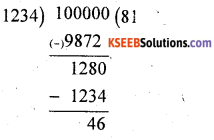KSEEB Solutions for Class 8 Maths Chapter 1 Playing with Numbers Additional Questions

Students can Download Maths Chapter 1 Playing with Numbers Additional Questions and Answers, Notes Pdf, KSEEB Solutions for Class 8 Maths helps you to revise the complete Karnataka State Board Syllabus and score more marks in your examinations.

Karnataka State Syllabus Class 8 Maths Chapter 1 Playing with Numbers Additional Questions

Question 1.
Choose the correct option.
(a) The general form of 456 is
A. (4 × 100) + (5 × 10) + (6 × l)
B. (4 × 100) + (6 × 10) + (5 × 1)
C. (5 × 100) + (4 × 10) + (6 × 1)
D. (6 × 100) + (5 × 10) + (4 × 1)
A. (4 × 100) + (5 × 10) + (6 × 1)

(b) Computers use
A. decimal system
B. binary system
C. base 5 system
D. base 6 system
B. binary system

(c) If $$\overline{a b c}$$ is a 3 digit number, then the number.
$$n=\overline{a b c}+\overline{a c b}+\overline{b a c}+\overline{b c a}+\overline{c a b}+\overline{c b a}$$ is always divisible by
A. 8
B. 7
C. 6
D. 5
C. 6

(d) If $$\overline{a b c}$$ is a 3-digit number then
$$n=\overline{a b c}-\overline{a c b}+\overline{b a c}-\overline{b c a}+\overline{c a b}-\overline{c b a}$$ is always divisible by
A. 12
B. 15
C. 18
D. 21
C. 18

(e) If IK × K1 = K2K, then the letter K stands for the digit.
A. 1
B. 2
C. 3
D. 4
A. 1

(f) The number 345111 is divisible by
A. 15
B. 12
C. 9
D. 3
D. 3

(g) The number of integers of the form 3AB4, where A, B denote some digits, which are divisible by 11 is
A. 0
B. 4
C. 7
D. 9
D. 9Question 2.
What is the smallest 5 digit number divisible by 11 and containing each of the digits 2, 3, 4, 5, 6?
The number is 24365
(∵ (2 + 3 + 5) – (4 + 6) = 0)

Question 3.
How many 5 – digit numbers divisible by 11 are there containing each of the digits 2, 3, 4, 5, 6?
The number having the digits 2, 3, 4, 5, 6 is divisible by 11 if 2, 3 and 5 are in the odd places and 4 and 6 are in the even places as (2 + 3 + 5) – (4 + 6) = 0 which is divisible by 11.

If 2 in the first place the numbers can be 24365, 26345, 24563, 26543 (4 numbers)

Similarly if 3 is in the first place we get 4 numbers and 5 is in the first place we get 4 numbers.
Totally there are 12 numbers.

Question 4.
If 49A and A49 where A > 0, have a common factor, find all possible values of A.
If A = 1 the numbers are 491 and 149 which do not have a common Tactor. If A = 2, the numbers are 492 and 249 which have 3 as a common factor.
If A = 3 the numbers are 493 and 349 which do not have a common factor.
If A = 4, the numbers are 494 and 449 which do not have a common factor.
If A = 5, the numbers are 495 and 549 which have a common factor 3.
If A = 6, the numbers are 496 and 649 8 which do not have a comon factor.
If A = 7 the numbers are 497 and 749 which have a common factor 7.
If A = 8, the numbers are 498 and 849 which have a common factor 3.
If A = 9, the numbers are 499 and 949 which do not have a. common factor,
∴ the values of K are 2, 5, 7 or 8.Question 5.
Write 1 to 10 using 3 and 5, each atleast once and using addition and subtraction
(For example 7 = 5 + 5 – 3)
1 = 3 + 3 – 5
2 = 5 – 3
3 = 5 + 3 – 5
4 = 3 + 3 + 3 – 5
5 = 3 + 5 – 3
6 = 5 + 5 + 5 – 3 – 3 – 3
7 = 5 + 5 – 3
8 = 5 + 3
9 = 5 + 5 + 5 – 3 – 3
10 = 5 + 5 + 3 – 3

Question 6.
Find all 2-digit numbers each of which is divisible by the sum of its digits.
The numbers are 10, 12, 18, 21,
24, 27, 36, 42, 45, 54, 63, 72 and 81.

Question 7.
The page numbers of a book written in a row gives a 216 digit number.
How many pages are there in the book?
Page numbers 1 to 9 written in a row form a 9 digit numbers. The page numbers 10 to 99 (= 90 pages) form 180 digit number.
The remaining number digits i.e., (216 – (9 + 180)) = 27
27 is formed by pages 100 to 108 (9 pages)
∴ The total number of pages = 9 + ’90 + 9 = 108Question 8.
Look at the following pattern.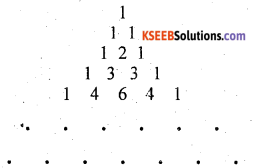This is called Pascal’s triangle. What is the middle number in the 9th row?
Complete the pascal’s triangle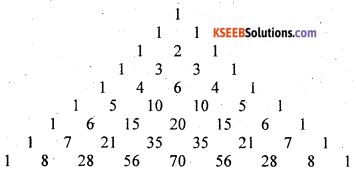The middle number in the 9th row is 70.

Question 9.
Complete the adjoining magic square (Hint. In a 3 × 3 magic square the magic sum is 3 . times the central number)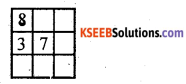Central number is 7. The magic sum is 3 × 7 = 21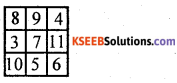Question 10.
Find all 3 digit natural numbers which are 12 times as large as the sum of their digits.
The number 108. Because 1 + 0 + 8 = 9 and 12 × 9 = 108Question 11.
Find all digits x, y such that $$\overline{34 \times 5 y}$$ is divisible by 36.
A number is divisible by 36 if it is divisible by both 4 and 9 as 4 × 9 = 36.
If the last two digits number is divisible by 4 then the number is divisible by 4. So in the given number y can be 2 or 6 as 52 and 56 are divisible by 4.

If the sum of all the digits of a number is divisible by 9 then the number is di-visible by 9. In the given number 34x5y if y = 2 and x = 4 the sum of the digits . 3 + 4 + 4 + 5 + 2 = 18 which is
divisible by 9

∴ 34452 is divisible by 4, 9 and 36
Similarly if y = 6 and x = 0 and y = 6 and x = 9 the number is divisible by 36

Question 12.
Can you divide the numbers 1, 2, 3, 4, 5, 6, 7, 8, 9, 10 into two groups
such that the product of numbers in one group divides the product of numbers in the other group and the quotient is minimum.
Group 1 = {1, 2, 3, 4, 5, 6, 7} the product is 1 x 2 x 3 x 4 x 5 x 6 x 7 = 5040
Group 2 = {8,9,10} the product is 8 × 9 × 10 = 720
$$\frac{\text { Group } 1}{\text { Group } 2}=\frac{5040}{720}=7$$Question 13.
Find all 8 digit numbers 273A49B5
which are divisible by 11 as well as 25.
The number is divisible by 25 if the number formed by last 2 digits is divisible by 5. In the number 273A49B5, B is either 2 or 7.
Let B is 2 then the number is divisible by 11 if (sum of oddly placed digits) (sum of evenly place ! digits) = multiple of 11
∴ (2 + 3 + 4 + 2) – (7 + A + 9 + 5) => 11 – (21 + A) = multiple of 11. It is possible when A = 1
∴ The number is 27314925.
Similarly if B = 7 then A must be 6
∴ The number is 27364975

Question 14.
Suppose a, b are integers such that 2 + a and 35 – b are divisible by 11 prove that a + b is divisible by 11.
2 + a and 35 – b
are divisible by 11
∴ 2 + a – (35 – b)
is also divisible by 11
2 + a – 35 + b = a + b – 33 is divisible by 11
∴ – 33 is divisible by 11 Hence a + b is divisible by 11Question 15.
In the multiplication table A8 x 3B = 2730, A and B represnet distinct digits different from 0. Find A + B.
A8 × 3B = 2730 The units place of the product is 0 B must be 5.
The product is A8 × 35. 35 × 8 = 280.
We write 0 in the units place and 28 in carried
∴ 35 x A + 28 = 273 ⇒ 35 x A = 273 – 28
= 245 => A = $$\frac{245}{35}$$ ⇒ A = 7
∴ A = 7 and B = 5
A + B = 7 + 5 = 12

Question 16.
Find the least natural number which leaves the remainder 6 and 8 when divided by 7 and 9 respectively.
Since 7 – 6 = 1, 9 – 8 = 1
The remainder in each case in less than the devisor by 1.
Hence if 1 is added to the required number it becomes exactly divisible by 7 and 9.

The least number divisible by 7 and 9 is_ in LCM of 7 and 9 which is equal to 63
The required number is 63 – 1 = 62Question 17.
Prove that the sum of the cubes of three consecutive natural numbers is always divisible by 3.
Let the 3 consecutive natural num-bers be x, x + 1 and x + 2.
Sum of their cubes
= x + (x + l)3 + (x + 2) 3= x3 + x3 + 13 + 3x(x +1) + x3 + 23 + 6x(x + 2)= x3 + x3 +1 + 3x2 + 3x + x3 + 8 + 6x2 + 12x= 3x3 + 9x2 +15x + 9 .= 3(x3 + 3x2 +5x + 3)
Sum of their cubes is a multiple of 3 which means that it is always divisible by 3.

Question 18.
What is the smallest number you have to add to 100000 to get a multiple of 1234?